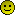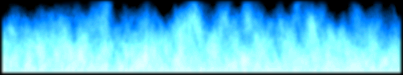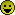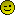###Author Topic: FreeBASIC TinyPTC extended graphics functionality lib.  (Read 1615 times)

0 Members and 1 Guest are viewing this topic.

####Blitz Amateur##### FreeBASIC TinyPTC extended graphics functionality lib.
« on: May 06, 2006 »
I'm working on an FB lib to mimic some of the drawing capabilities of BB. You'll have to tell me how you think it's going so far =)

http://www.geocities.com/dantthegreat/TinyPTCLib.zip

I couldn't just upload it as .bi, so it's zipped.For right now, it can do plotting, and lines, and open a graphics display and clear it and flip it to the screen. It also uses a pointer to reference the screen buffer, so that size can be allocated. I'm not sure how FB handles memory that is allocated, but not specifically freed. So there may be a memory leak. It should be easy enough to get rid of though####Shockwave

• good/evil
• Founder Member
••• Posts: 17378
• Karma: 497
• evil/good##### Re: FreeBASIC TinyPTC extended graphics functionality lib.
« Reply #1 on: May 06, 2006 »
That's a great idea, it's something that everyone needs to have really.
I've been working on a few things like this myself, so far I can plot dots hehe! Still, everything is made up of pixels.

####Clyde

• A Little Fuzzy Wuzzy
••• Posts: 7271
• Karma: 71##### Re: FreeBASIC TinyPTC extended graphics functionality lib.
« Reply #2 on: May 08, 2006 »
Coolness and very nice idea BACheers and all the best,
Clyde

####Blitz Amateur##### Re: FreeBASIC TinyPTC extended graphics functionality lib.
« Reply #3 on: June 09, 2006 »
An update. My linedraw was slightly borked, so I fixed it and optimised a bitCode: [Select]
`#Include "tinyptc.bi"'TinyPTC Graphics Interface Lib''Feel free to use this in anything you like, you can credit if you like, but not required. This is just something I wrote so people can do TinyPTC stuff easier =)''This Library takes the basic functionality of TinyPTC and allows more advanced'drawing operations with simplified commands. Commands such as Graphics,'FlipSurf, ClsSurf, Plot, ColorSet, and DrawLine''''Command reference is coming soon, until then, commands should be fairly simply'Understood. Redirect any questions either to "Http://www.dbfinteractive.com"'Or to blitzamateur@yahoo[dot]comDim shared as integer ptr bufferdim shared as integer GFXWID, GFXHT, GFXWIDH, GFXHTH, CurColor, CLEARCOUNTdeclare sub Graphics(ByVal wid as integer, ByVal ht as integer, Byval appname as string)declare sub FlipSurf()declare sub ClsSurf()declare sub Plot(ByVal x as integer, ByVal y as integer)declare sub ColorSet(ByVal r as integer, ByVal g as integer, ByVal b as integer) declare sub DrawLine(ByVal x1 as single, ByVal y1 as single, ByVal x2 as single, ByVal y2 as single)declare sub Oval(ByVal x as single, ByVal y as single, ByVal w as single, ByVal h as single)Sub Graphics(ByVal Wid as integer, ByVal Ht as integer, ByVal AppName as string="FB w/ TinyPTC")        if ptc_open(AppName, Wid, Ht) = 0 then        print "Cannot open graphics display at "+str(wid)+", "+str(ht)        sleep        end -1    endif    buffer = callocate(wid*ht, Len(integer))    CLEARCOUNT = wid*ht*Len(integer)    GFXWID = Wid    GFXHT = Ht    GFXWIDH = GFXWID shr 1    GFXHTH = GFXHT shr 1    End SubSub FlipSurf()        ptc_update(buffer)    End SubSub ClsSurf()        clear *buffer, 0, CLEARCOUNT    End SubSub Plot(ByVal x as integer, ByVal y as integer)        buffer[(y*GFXWID+x)] = CurColor    End SubSub ColorSet(ByVal r as integer, ByVal g as integer, ByVal b as integer)        CurColor = ( r shl 16 ) + ( g shl 8 ) + b    End SubSub Oval (ByVal x as single, ByVal y as single, ByVal w as single, ByVal h as single)    End Sub    Sub DrawLine(ByVal x1 as single,ByVal y1 as single,ByVal x2 as single,ByVal y2 as single)    dim temp as single    dim xd as double    dim yd as double    dim y as double    dim ty as double    dim x as double    dim tx as double    dim xs as double    dim ys as double    'if x2 < x1 then        xd = abs(x1-x2)    'else    '    xd = abs(x2-x1)    'endif    'if y2 < y1 then        yd = abs(y1-y2)    'else    '    yd = abs(y2-y1)    'endif    if xd > yd then        if x2 < x1 then            temp = x1            x1 = x2            x2 = temp            temp = y1            y1 = y2            y2 = temp        endif        xd = x2-x1        yd = y2-y1        ys = yd/xd        y=y1        for x=x1 to x2            buffer[cint(y+.49)*GFXWID+cint(x+.49)] = CurColor            y=y+ys        next    else        if y2 < y1 then            temp = x1            x1 = x2            x2 = temp            temp = y1            y1 = y2            y2 = temp        endif        xd = x2-x1        yd = y2-y1        xs = xd/yd        x=x1        for y=y1 to y2            buffer[cint(y+.49)*GFXWID+cint(x+.49)] = CurColor            x = x + xs        next    endifend sub`